The equations in Sec. 5.10 are combined using algorithms that couple the solutions for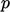and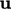. One algorithm is SIMPLE (Semi-Implicit Method for Pressure-Linked Equations)3, which is presented here with a modern interpretation.

The SIMPLE algorithm is generally used to produce steady ﬂow solutions in CFD. These solutions are directly applicable for ﬂows that reach a steady state, i.e. when ﬂow variables stop changing in time. They can also provide approximate solutions to ﬂows that are moderately unsteady, usually at a lower cost than a more exact transient solution.

An example of the algorithm is shown for the system of equations presented in Sec. 5.9 . The time derivative (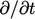) terms are omitted due to the steady-state assumption.

The algorithm involves an iterative sequence with steps,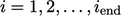. It begins by constructing a matrix equation for energy which is under-relaxed by a factor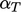. The equation is solved for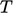, which is used to update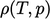according to an equation of state. A matrix equation is then constructed using all the terms from the momentum equation excluding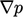, i.e.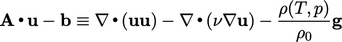(5.21)
The matrix equation is under-relaxed by a factor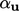before equating with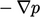and solving for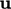(the momentum predictor).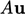and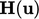are then evaluated from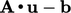(the momentum matrix), as described on page 351. They are used to form the pressure equation, which is solved for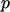.

The new pressureis used to correct the ﬂux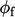so that it obeys mass conservation better (the ﬂux corrector). It is then under-relaxed by a factor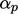before correctingbefore the next solution step begins (the momentum corrector).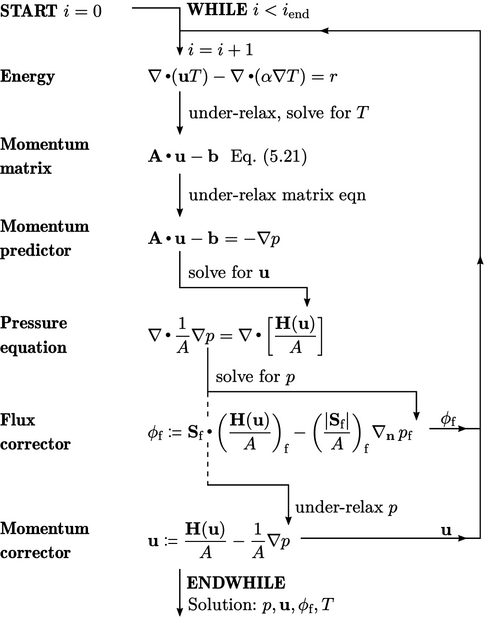3Suhas Patankar and Brian Spalding, A calculation procedure for heat, mass and momentum transfer in three-dimensional parabolic ﬂows, 1972.

Notes on CFD: General Principles - 5.12 Steady-state solution